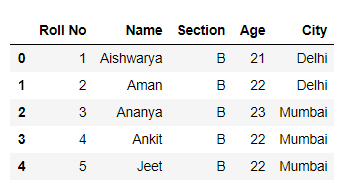Open In App

# How to skip rows while reading csv file using Pandas?

Python is a good language for doing data analysis because of the amazing ecosystem of data-centric python packages. Pandas package is one of them and makes importing and analyzing data so much easier.

Here, we will discuss how to skip rows while reading csv file. We will use read_csv() method of Pandas library for this task.

Syntax: pd.read_csv(filepath_or_buffer, sep=’, ‘, delimiter=None, header=’infer’, names=None, index_col=None, usecols=None, squeeze=False, prefix=None, mangle_dupe_cols=True, dtype=None, engine=None, converters=None, true_values=None, false_values=None, skipinitialspace=False, skiprows=None, nrows=None, na_values=None, keep_default_na=True, na_filter=True, verbose=False, skip_blank_lines=True, parse_dates=False, infer_datetime_format=False, keep_date_col=False, date_parser=None, dayfirst=False, iterator=False, chunksize=None, compression=’infer’, thousands=None, decimal=b’.’, lineterminator=None, quotechar='”‘, quoting=0, escapechar=None, comment=None, encoding=None, dialect=None, tupleize_cols=None, error_bad_lines=True, warn_bad_lines=True, skipfooter=0, doublequote=True, delim_whitespace=False, low_memory=True, memory_map=False, float_precision=None)

Some useful parameters are given below :

Method 1: Skipping N rows from the starting while reading a csv file.

Code:

## Python3

 `# Importing Pandas library``import` `pandas as pd` `# Skipping 2 rows from start in csv``# and initialize it to a  dataframe``df ``=` `pd.read_csv(``"students.csv"``,``                  ``skiprows ``=` `2``)` `# Show the dataframe``df`

Output :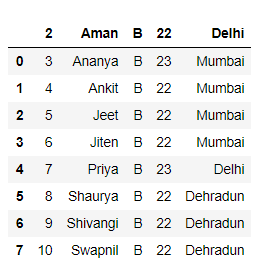Method 2: Skipping rows at specific positions while reading a csv file.

Code:

## Python3

 `# Importing Pandas library``import` `pandas as pd` `# Skipping rows at specific position``df ``=` `pd.read_csv(``"students.csv"``,``                  ``skiprows ``=` `[``0``, ``2``, ``5``])` `# Show the dataframe``df`

Output :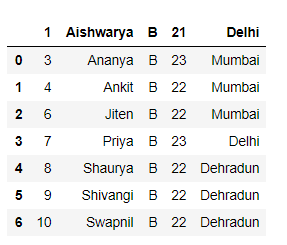Method 3: Skipping N rows from the starting except column names while reading a csv file.

Code:

## Python3

 `# Importing Pandas library``import` `pandas as pd` `# Skipping 2 rows from start``# except the column names``df ``=` `pd.read_csv(``"students.csv"``,``                 ``skiprows ``=` `[i ``for` `i ``in` `range``(``1``, ``3``) ])` `# Show the dataframe``df`

Output :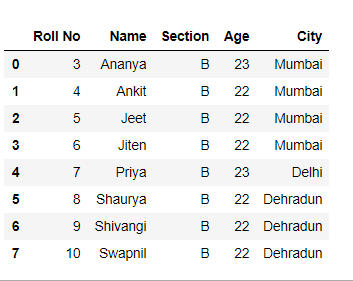Method 4: Skip rows based on a condition while reading a csv file.

Code:

## Python3

 `# Importing Pandas library``import` `pandas as pd` `# function for checking and``# skipping every 3rd line``def` `logic(index):` `    ``if` `index ``%` `3` `=``=` `0``:``        ``return` `True` `    ``return` `False` `# Skipping rows based on a condition``df ``=` `pd.read_csv(``"students.csv"``,``                 ``skiprows ``=` `lambda` `x: logic(x) )` `# Show the dataframe``df`

Output :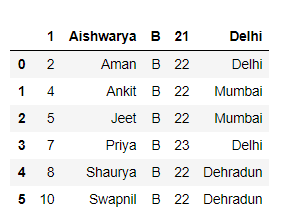Method 5: Skip N rows from the end while reading a csv file.

Code:

## Python3

 `# Importing Pandas library``import` `pandas as pd` `# Skipping 2 rows from end``df ``=` `pd.read_csv(``"students.csv"``,``                  ``skipfooter ``=` `5``,``                  ``engine ``=` `'python'``)` `# Show the dataframe``df`

Output :